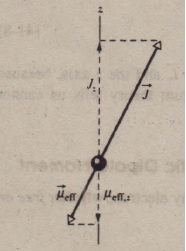# Orbital and Spin Angular Momenta Combined

Orbital and Spin Angular Momenta Combined

.which is the vector sum of the angular momenta of the individual electronsboth0 their orbital and their spin angular momenta. The number of electrons (and the number of protons) in a neutral atom is the atomic number (or charge number) Z. Thus. for a neutral atom.Similarly, the total magnetic dipole moment of the multielectron atom is the vector sum of the magnetic dipole moments (both orbital and spin) of its individual electrons. However. because of the factor of 2 i. the resultant magnetic dipole moment for the atom does not have the direction of the vector -7: instead, it makes a certain angle with that vector. The effective magnetic dipole moment icier for the atom is the component oi the vector sum of the individual magnetic  dipole moments in the direction of – J ).As you will see in the next section, in typical atoms the orbital angular momenta and the spin angular momenta of most of the electrons sum vectorially to zero. Then 7and ii.tr of those atoms are due to a relatively small number of electrons, often only a single valence electron.CHECKPOINT 1: An electron in a quantum staleble for which the magnitude of the electron’s orbital angular momentum Lis 2J31t. How many projections of the electron’s orbital magnetic dipole meant on a : axis are allowed? According to classical physics, the components 1.1.: of silver atoms passing through the magnetic field in should range in value from – J.L (the dipole moment it is directed straight down the z axis) to +J.L (it is directed straight up the  z axis). Thus, from , there should be a range of forces on the atoms, and e a range of deflections of the atoms, from a greatest downward deflection  ‘to a greatest upward deflection. This means that we should expect the atoms to land along a vertical line on the glass plate. However, this does not happen